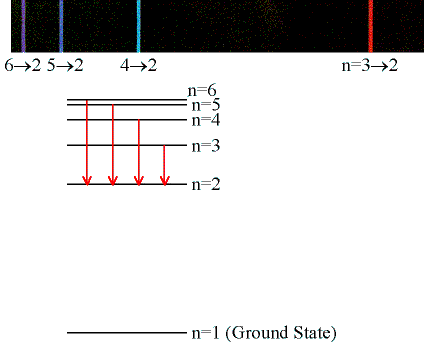# Question #d0cfc

Jun 29, 2016

Here's how you could do it:

#### Explanation:

It seems you need to produce a calibration curve for the distance measured against the wavelength of the emission line using Excel.

I got this from your data:I assumed cm but I guess it could be mm - you should put this on your table.

Point 28 is an outlier - you should repeat this if you can.

I then used the 3 lines from the hydrogen lamp to read off the values of the wavelength.

To do this enter your data in a table and make a scatter chart. Click on a data point and "add trend line". You can select "linear" and it will give you the line of best fit through the points.

You can select "display ${\text{R}}^{2}$ value" as asked and also "display equation" which returns the equation of the straight line.

The ${\text{R}}^{2}$ value is the square of the correlation coefficient for which I got $0.8747$.

This means the correlation coefficient $= \sqrt{0.8747} = 0.935$ which is very close to an exact correlation of $1$ - though I chose a binomial trend line.

I have manually read the wavelengths off the chart, though you could use the equation given.

So for red:

$d = 33.1 \text{cm}$ and $\lambda = 659.4 \text{nm}$

This is an experimental value. You are asked to compare this with the calculated value using the Rydberg Equation:

$\frac{1}{\lambda} = R \left[\frac{1}{n} _ {1}^{2} - \frac{1}{n} _ {2}^{2}\right]$

$R = 1.0974 \times {10}^{7} {\text{ ""m}}^{- 1}$

The Balmer Series, which you are dealing with, involves transitions down to the $n = 2$ energy level:The red emission line will be the lowest in energy so will have the longest wavelength corresponding to $n = 3 \rightarrow 2$ .

Putting this into the Rydberg Expression:

$\frac{1}{\lambda} = 1.0974 \times {10}^{7} \left[\frac{1}{2} ^ 2 - \frac{1}{3} ^ 2\right]$

(You made a mistake in your annotation and wrote $\frac{1}{2}$ instead of $\frac{1}{2} ^ 2$ )

$\therefore \frac{1}{\lambda} = 0.1524 \times {10}^{7} {\text{ ""m}}^{- 1}$

$\therefore \lambda = 656.1 \text{ ""nm}$

So we have:

Experimental: $659.4 \text{ ""nm}$

Theoretical: $656.1 \text{ ""nm}$

That's reasonably good. You can work out the difference as a percentage as asked for in your error calculation.

Then work out $4 \rightarrow 2$ and $5 \rightarrow 2$ in the same way.